# Motion Distance Displacement Speed average and instantaneous Velocity

• Slides: 18Motion • • • Distance Displacement Speed: average and instantaneous Velocity Acceleration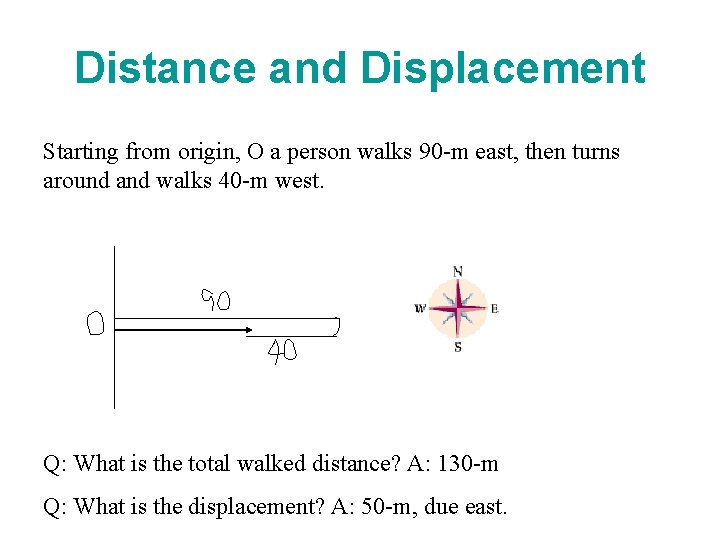Distance and Displacement Starting from origin, O a person walks 90 -m east, then turns around and walks 40 -m west. Q: What is the total walked distance? A: 130 -m Q: What is the displacement? A: 50 -m, due east.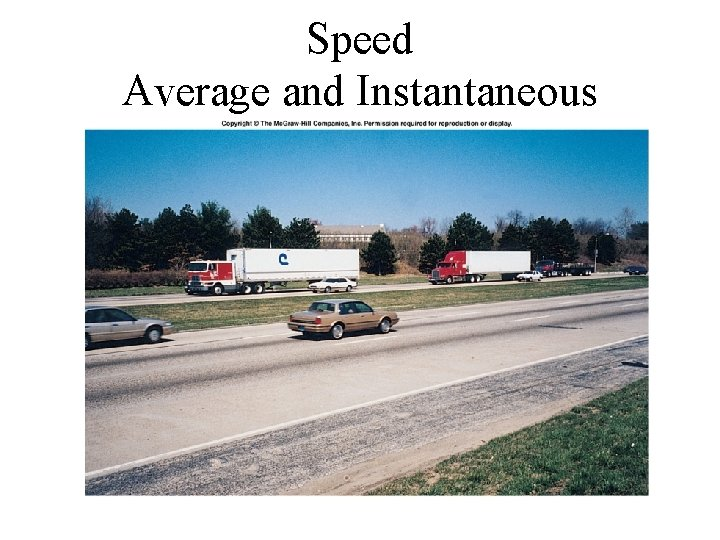Speed Average and InstantaneousLet’s Go to Myrtle BeachAverage Speed Average speed equals the distance traveled divided by the time of travel. Speed is a scalar quantity. Unit of speed: m/s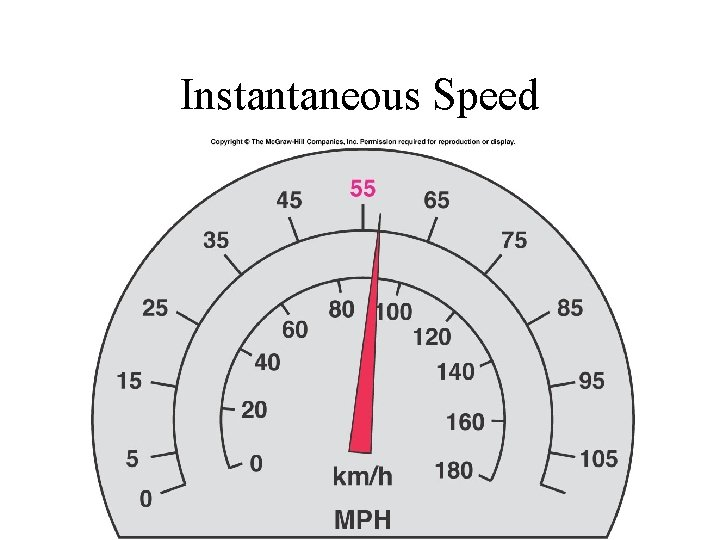Instantaneous Speed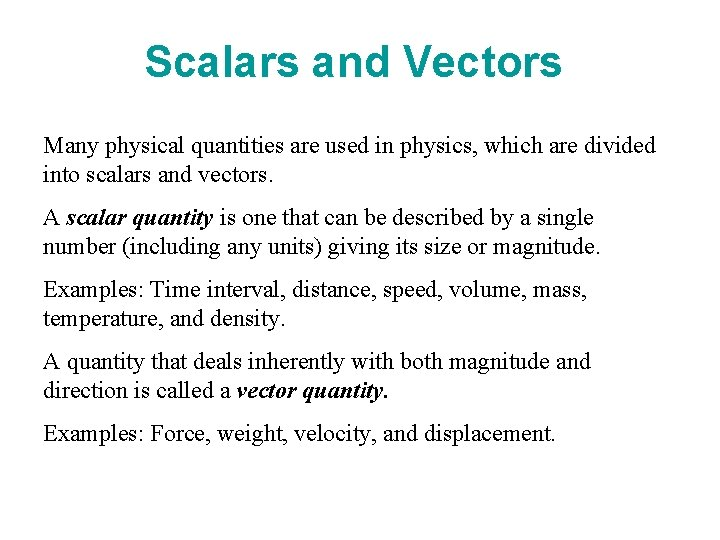Scalars and Vectors Many physical quantities are used in physics, which are divided into scalars and vectors. A scalar quantity is one that can be described by a single number (including any units) giving its size or magnitude. Examples: Time interval, distance, speed, volume, mass, temperature, and density. A quantity that deals inherently with both magnitude and direction is called a vector quantity. Examples: Force, weight, velocity, and displacement.Scalars and Vectors Scalar Vector Distance Displacement Speed Velocity Mass Weight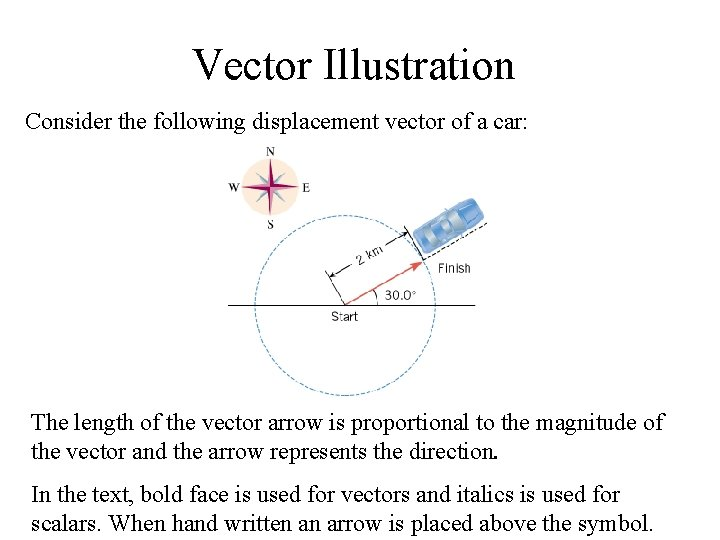Vector Illustration Consider the following displacement vector of a car: The length of the vector arrow is proportional to the magnitude of the vector and the arrow represents the direction. In the text, bold face is used for vectors and italics is used for scalars. When hand written an arrow is placed above the symbol.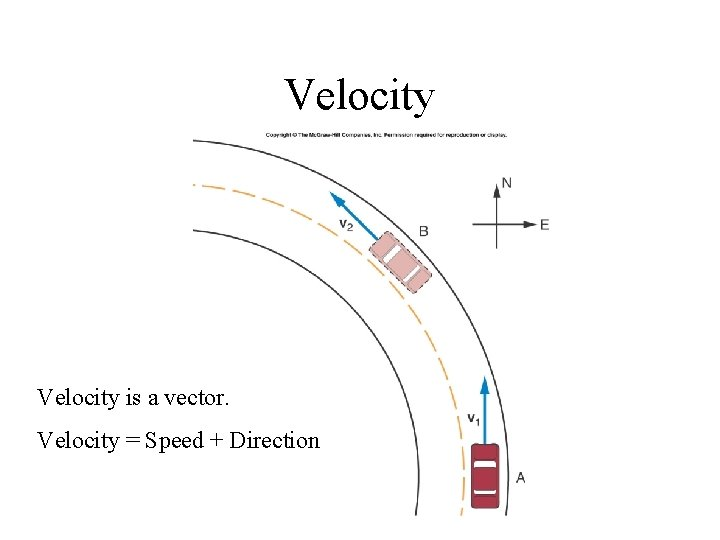Velocity is a vector. Velocity = Speed + Direction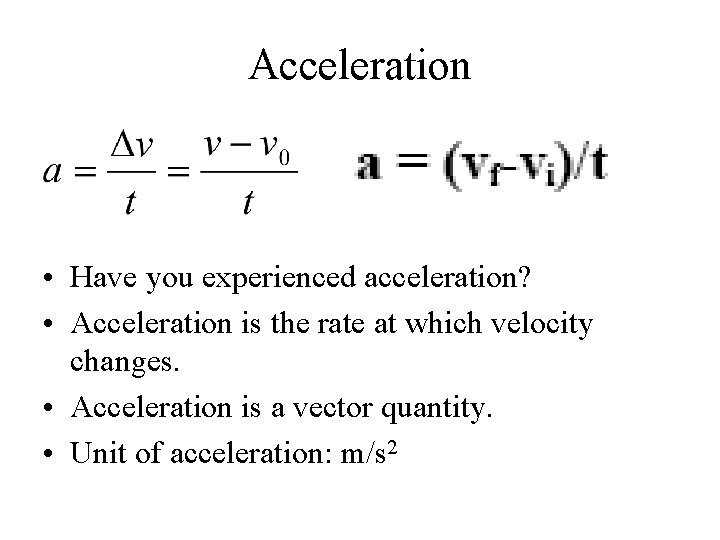Acceleration • Have you experienced acceleration? • Acceleration is the rate at which velocity changes. • Acceleration is a vector quantity. • Unit of acceleration: m/s 2AccelerationAcceleration can be Positive or Negative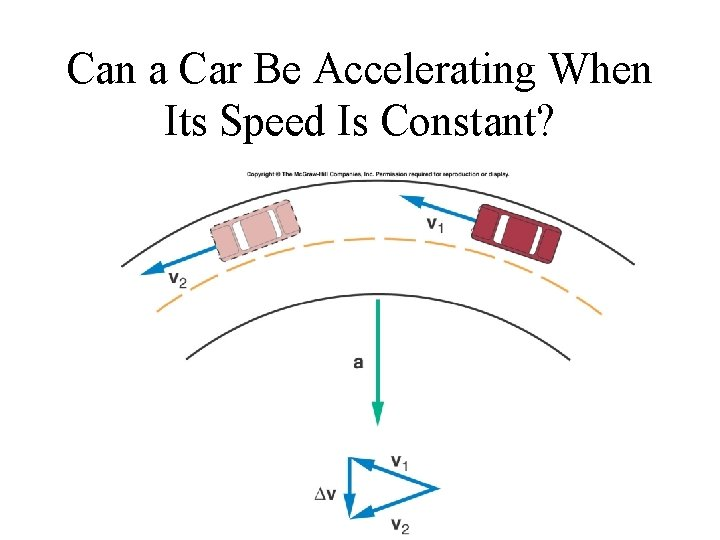Can a Car Be Accelerating When Its Speed Is Constant?Acceleration of a Sports Car • http: //www. cars. com/go/crp/buying. Guides/Story. jsp? section=Spor ts&story=sports. Quickest&subject=stories&referer=&year=2005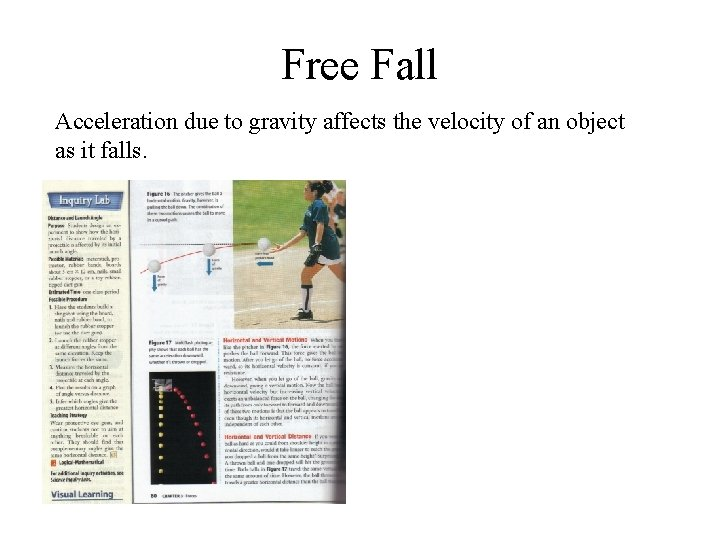Free Fall Acceleration due to gravity affects the velocity of an object as it falls.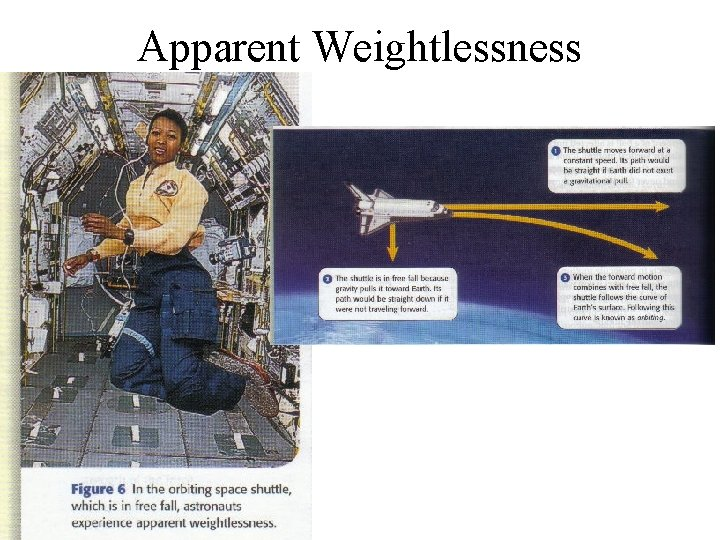Apparent Weightlessness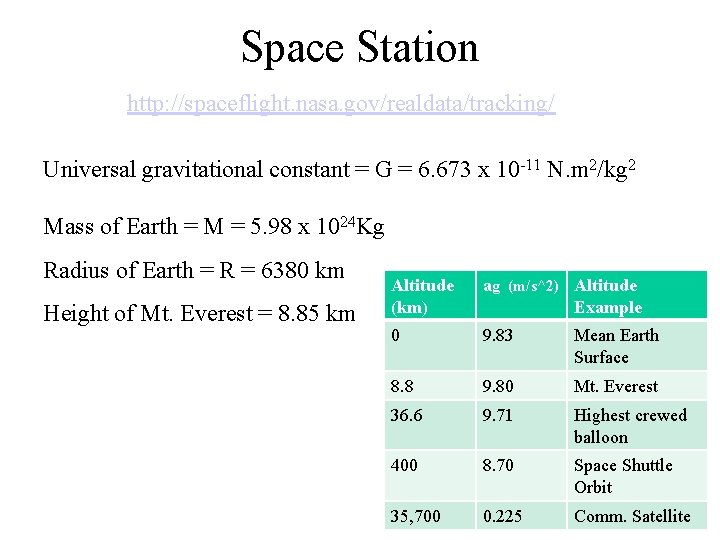Space Station http: //spaceflight. nasa. gov/realdata/tracking/ Universal gravitational constant = G = 6. 673 x 10 -11 N. m 2/kg 2 Mass of Earth = M = 5. 98 x 1024 Kg Radius of Earth = R = 6380 km Height of Mt. Everest = 8. 85 km Altitude (km) ag (m/s^2) Altitude Example 0 9. 83 Mean Earth Surface 8. 8 9. 80 Mt. Everest 36. 6 9. 71 Highest crewed balloon 400 8. 70 Space Shuttle Orbit 35, 700 0. 225 Comm. Satellite